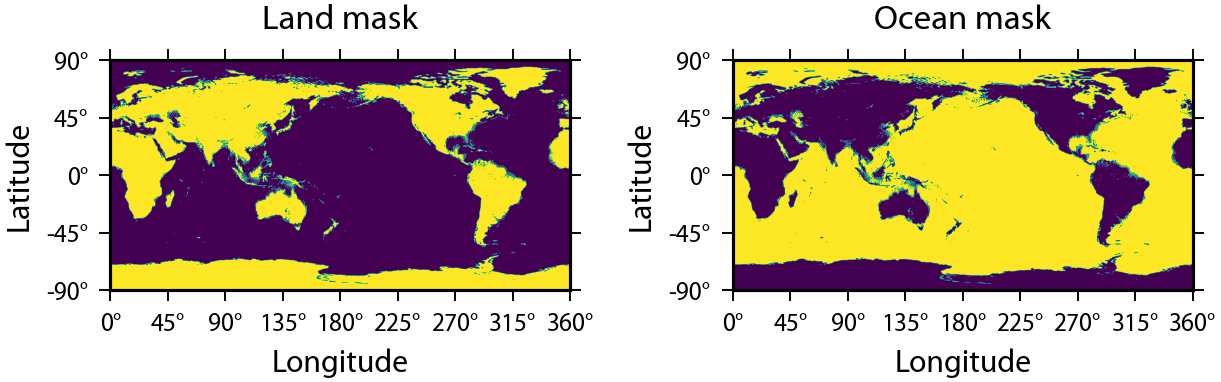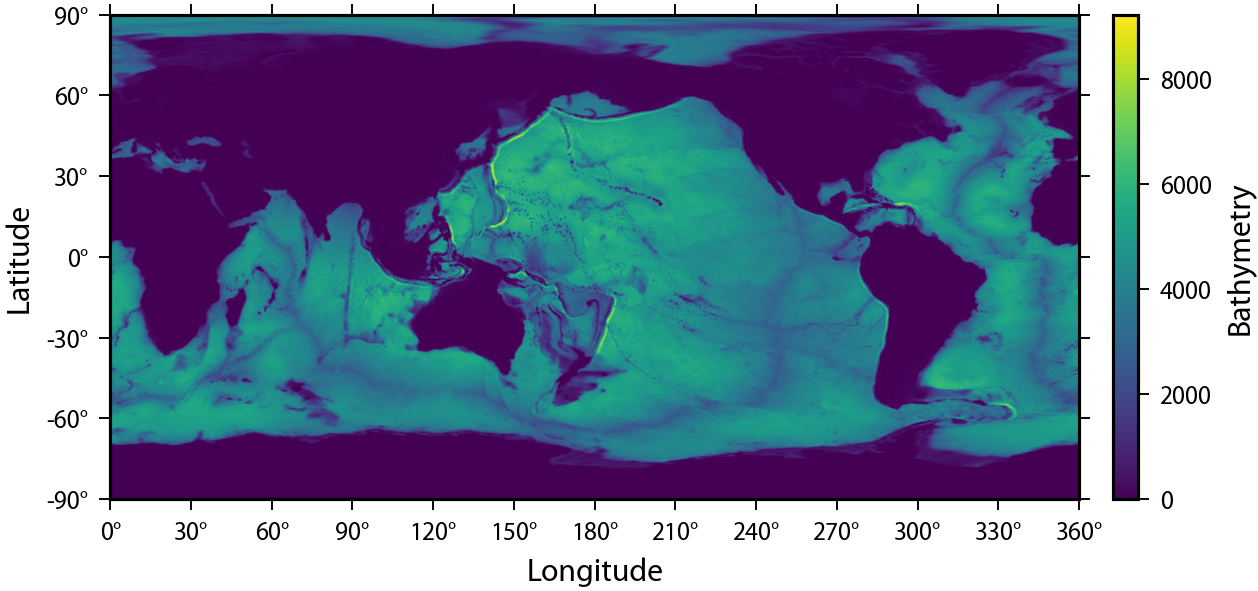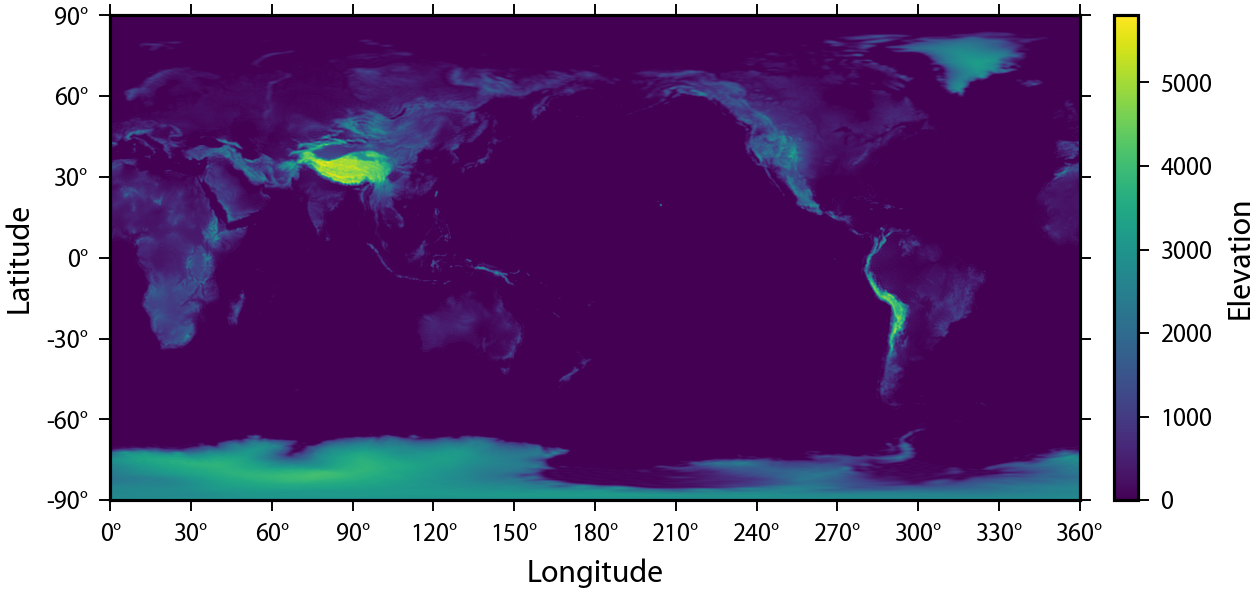# Advanced usage of the SHWindow class interface¶

The SHWindow class provides an interface to compute window functions that concentrate energy spatially in a given region and within a given spherical harmonic bandwidth. This allows to calculate spectral estimates localized to specific regions with the best possible spectral resolution.

To demonstrate, in this example, we will determine the spectrum of the bathymetry of Earth and compare it with that of the surface topography. To this end, we will need to generate window functions that are separately concentrated over the land and the oceans. To start, we read the topography of Earth from the example files, create masks for the oceans and land, and plot the masks:

In :
%matplotlib inline

import matplotlib.pyplot as plt
import numpy as np
import pyshtools as pysh

In :
pysh.utils.figstyle(rel_width=0.75)
%config InlineBackend.figure_format = 'retina'  # if you are not using a retina display, comment this line

In :
clm = pysh.datasets.Earth.Earth2014.tbi(lmax=360)
topo = clm.expand(grid='DH2')

land_mask = pysh.SHGrid.from_array(topo.data > 0)
ocean_mask = pysh.SHGrid.from_array(topo.data <= 0)

fig, (col1, col2) = plt.subplots(1, 2)
land_mask.plot(ax=col1, tick_interval=(45, 45), minor_tick_interval=None)
col1.set(title='Land mask')
ocean_mask.plot(ax=col2, tick_interval=(45, 45), minor_tick_interval=None)
col2.set(title='Ocean mask');
fig.tight_layout()Next, let's plot the masked topography over the oceans and land:

In :
fig, ax = (topo * ocean_mask *(-1)).plot(colorbar='right', cb_label='Bathymetry', show=False)    # show=False is used to avoid a warning when plotting in inline mode
fig2, ax2 = (topo * land_mask).plot(colorbar='right', cb_label='Elevation', show=False)## Optimally concentrated window functions¶

SHTOOLS provides a method to solve the so called concentration problem, that is, to find windows with a specified maximum spherical harmonic degree lmax that are optimally concentrated in the region of interest. The smaller the region, the larger lmax will need to be to allow for a good concentration. Because the oceans span a larger area than the land mass, the spherical harmonic bandwidth of the ocean windows lmax_ocean can be smaller than the spherical harmonic bandwidth of the land windows lmax_land while still providing the same number of well concentrated windows.

Creating localization windows is easy using the SHWwindow method from_mask(). All we need to do is provide the mask, the maximum spherical harmonic bandwidth, and optionally, the number of windows to return:

In :
lmax_ocean = 9
lmax_land = 21
nwins = 10

ocean_windows = pysh.SHWindow.from_mask(ocean_mask.to_array(), lmax_ocean, nwin=nwins)
land_windows = pysh.SHWindow.from_mask(land_mask.to_array(), lmax_land, nwin=nwins)


We can find out information about the windows that were created using the info() method, and the windows can be plotted using the plot_windows() method. In plotting the windows, the loss factor of the window is provided above each image, which is the proportion of the energy of the window that lies outside the region of interest. This is simply 1 minus the concentration factor. For our example, it is seen that in order to obtain 10 well localized windows with loss factors of less than 0.1%, the spectral bandwidth of the land windows needs to be more than twice as large as that of the ocean windows.

In :
ocean_windows.info()
fig, ax = ocean_windows.plot_windows(nwins, maxcolumns=2, show=False)

kind = 'mask'
lwin = 9
nwin = 10
shannon = 70.645322
area (radians) = 8.877553e+00
Taper weights are not set